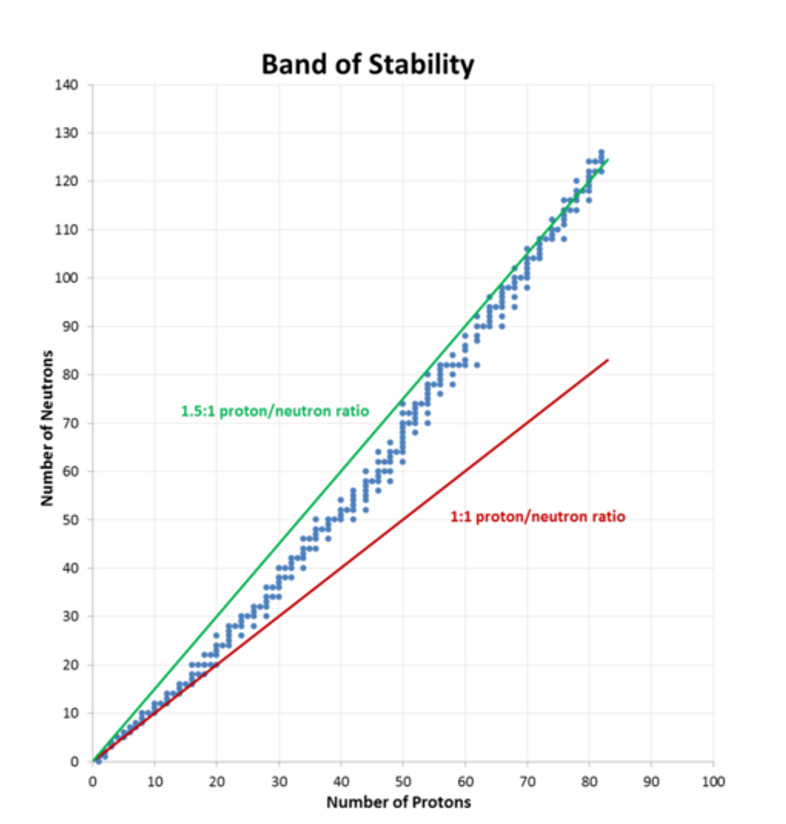This is part of preliminary HSC Chemistry course under the topic of atomic structure and atomic mass.

### HSC Chemistry Syllabus

• Investigate the properties of unstable isotopes using natural and human-made radioisotopes as examples including but not limited to:
• Types of balanced nuclear reactions

• There are three types of radiation: Alpha (\alpha), Beta (\beta), and Gamma (\gamma)
 Property Alpha (α) Beta (β) Gamma (ɣ) Component Helium nucleus Electron Electromagnetic Radiation Nuclear Symbol $$^4_2\alpha$$ $$^0_{-1}\beta$$ $$^0_0\gamma$$ Electric charge +2 -1 0 Penetration Poor penetration Stopped by thin paper Medium/low penetration Stopped by 5mm of aluminium Very strong penetration can travel through 2 inches of lead Velocity V = 0.05c V = 0.99c V = c

### Nuclear Reactions

Nuclear chemistry is the study of reactions that involve changes in nuclear structure. The term "nuclide" is used when referring to a specific atom of a nucleus and is denoted by the name of an element followed by a hyphen and its mass number. For example, a nuclide with 6 protons and 8 electrons would be named carbon-14.

Nuclear decay, also known as "radioactive decay", is a phenomenon which occurs when unstable nuclei emit radiation to transition to a more stable state. In the process of nuclear decay, the resulting daughter nuclei which are produced as a result of radioactive emission, have lower mass and lower energy (more stable).

Parent Nucleus \rightarrow Radiation + Daughter Nucleus

Like chemical equations where the number and types of atoms are accounted for to present a balanced chemical equation, nuclear decay equations can also be balanced. In this case, we can use the number and type of nucleons (proton or neutron) present to write a balanced nuclear equation for a nuclear decay reaction.

Example: element X undergoes alpha decay to produce element Y

 Parent nucleus $$\rightarrow$$ Radiation + Daughter nucleus $$^A_ZX$$ $$\rightarrow$$ $$^4_2\alpha$$ + $$^{A-4}_{Z-2}X$$ Radium-266 $$\rightarrow$$ Alpha Particle + Radon-222 $$^{266}_{88}Ra$$ $$\rightarrow$$ $$^4_2\alpha$$ + $$^{262}_{86}Ra$$

Example: element X undergoes beta decay to produce element Y

 Parent nucleus $$\rightarrow$$ Radiation + Daughter nucleus $$^A_ZX$$ $$\rightarrow$$ $$^0_{-1}\beta$$ + $$^{A}_{Z+1}X$$ Iodine-131 $$\rightarrow$$ Beta Particle + Xenon-131 $$^{131}_{53}I$$ $$\rightarrow$$ $$^0_{-1}\beta$$ + $$^{131}_{54}Ra$$

We previously discussed an isotope of carbon; carbon-12. However, carbon also exists in other isotopic forms such as carbon-13 and carbon-14 which differ in the number of neutrons they possess. A characteristic which distinguishes between these isotopes of carbon is that while carbon-12 is stable, both carbon-13 and carbon-14 are radioactive.

These radioactive isotopes, also known as radioisotopes, can be detected using either a Geiger counter or photographic film. While elements with an atomic number greater than 82, such as uranium, are all radioactive, for some elements such as rubidium and rhenium, only one or a few isotopes are radioactive

### Band of Stability

A nucleus is described as being ‘stable’ if it cannot be transformed into another isotope without an external input of energy. Of the thousands of nuclides (any form of atom that exists for a period of time) that exist, only about 250 are considered stable. In general, the number of neutrons required to stabilise a nucleus increases more rapidly than the number of protons shown in the graph and thus the neutron-to-proton ratio which is required for stable nuclei increases with increasing atomic number.

The graph below shows a narrow band which depicts an area known as the ‘band of stability’. This band ends at element 82 (bismuth), and from there all elements with proton number greater than or equal to 83 are radioactive. The reason why larger nuclei require larger numbers of neutrons to help make them stable is because their larger nuclei have more proton-proton repulsions. These protons which are positively charged, repel according to the well-known rule “opposites attract, similar repel”. Thus, to overcome this issue, the nuclei require larger numbers of neutrons to provide compensating strong forces to overcome these ‘electrostatic repulsions’ and hold the nucleus togetherThe kind of radioactive decay that a particular radionuclide undergoes depends to a large extent on its neutron-to-proton ratio compared to those of nearby nuclei within the belt of stability. There are two scenarios in which nuclide will decay

1. Nuclei with a neutron-to-proton ratio which is higher than the band of stability
• These neutron-rich nuclei can lower their ratio and move toward the band of stability via the emission of a beta particle. In beta decay, the neutron emits an electron and ‘sheds’ its negative electrical charge to turn into a proton.

1. Nuclei atomic number greater than or equal to 84
• These heavy nuclei which lie beyond the upper right edge of the band of stability will tend to undergo alpha emission to decrease both the number of neutrons and number of protons, significantly moving the nucleus diagonally toward the band of stability. In alpha decay, an alpha particle (a helium nucleus) which is composed of two protons and two neutrons is emitted